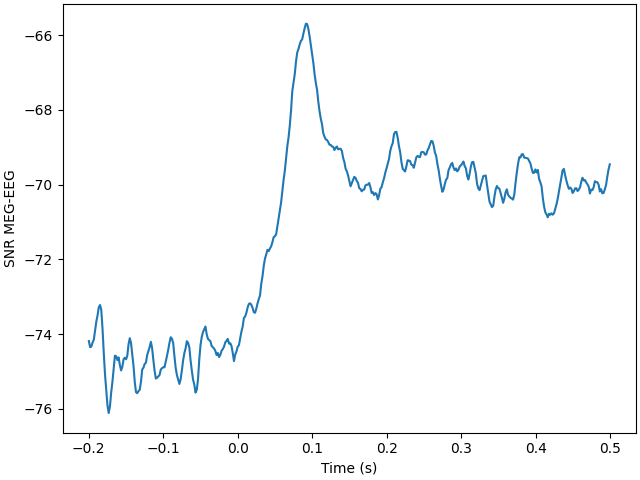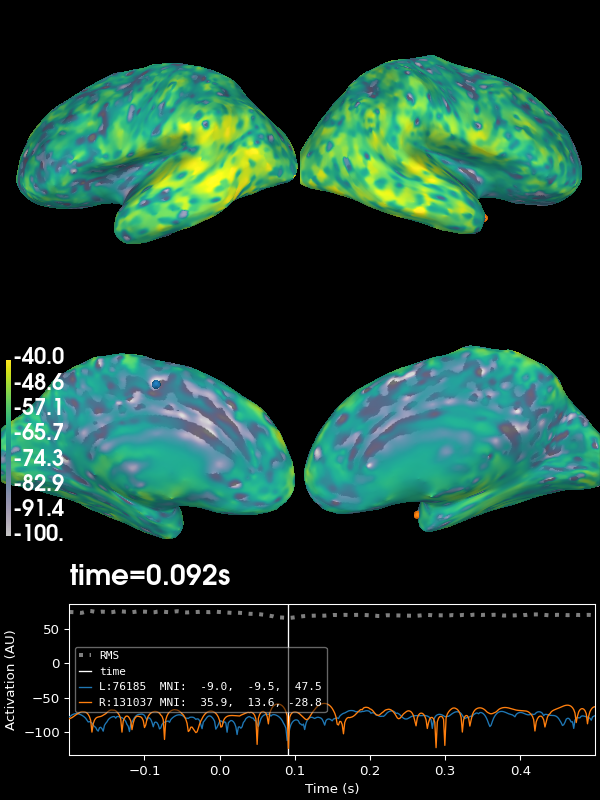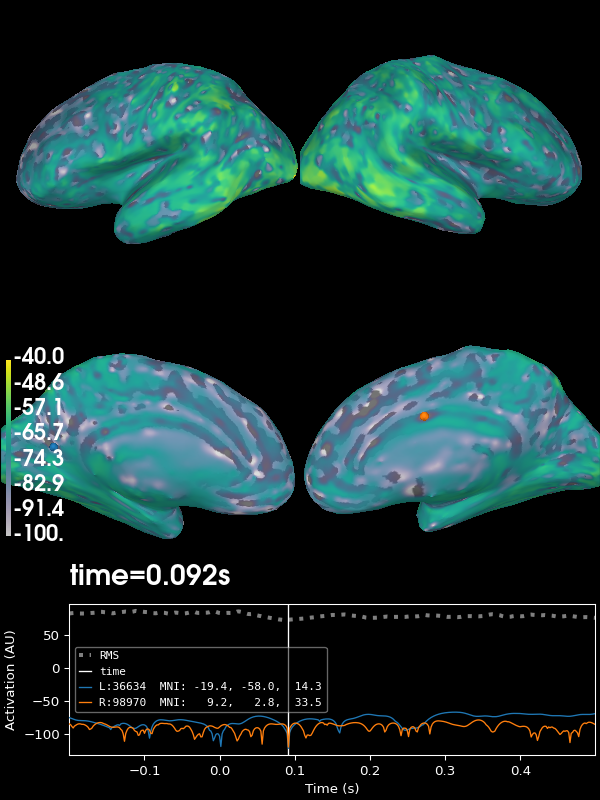# Computing source space SNR#

This example shows how to compute and plot source space SNR as in .

```# Author: Padma Sundaram <tottochan@gmail.com>
#         Kaisu Lankinen <klankinen@mgh.harvard.edu>
#
```
```import mne
from mne.datasets import sample
from mne.minimum_norm import make_inverse_operator, apply_inverse
import numpy as np
import matplotlib.pyplot as plt

print(__doc__)

data_path = sample.data_path()
subjects_dir = data_path / 'subjects'

meg_path = data_path / 'MEG' / 'sample'
fname_evoked = meg_path / 'sample_audvis-ave.fif'
baseline=(None, 0))
fname_fwd = meg_path / 'sample_audvis-meg-eeg-oct-6-fwd.fif'
fname_cov = meg_path / 'sample_audvis-cov.fif'

inv_op = make_inverse_operator(evoked.info, fwd, cov, fixed=True, verbose=True)

# Calculate MNE:
snr = 3.0
lambda2 = 1.0 / snr ** 2
stc = apply_inverse(evoked, inv_op, lambda2, 'MNE', verbose=True)

# Calculate SNR in source space:
snr_stc = stc.estimate_snr(evoked.info, fwd, cov)

# Plot an average SNR across source points over time:
ave = np.mean(snr_stc.data, axis=0)

fig, ax = plt.subplots()
ax.plot(evoked.times, ave)
ax.set(xlabel='Time (s)', ylabel='SNR MEG-EEG')
fig.tight_layout()

# Find time point of maximum SNR
maxidx = np.argmax(ave)

# Plot SNR on source space at the time point of maximum SNR:
kwargs = dict(initial_time=evoked.times[maxidx], hemi='split',
views=['lat', 'med'], subjects_dir=subjects_dir, size=(600, 600),
clim=dict(kind='value', lims=(-100, -70, -40)),
transparent=True, colormap='viridis')
brain = snr_stc.plot(**kwargs)
``````Reading /home/circleci/mne_data/MNE-sample-data/MEG/sample/sample_audvis-ave.fif ...
Read a total of 4 projection items:
PCA-v1 (1 x 102) active
PCA-v2 (1 x 102) active
PCA-v3 (1 x 102) active
Average EEG reference (1 x 60) active
Found the data of interest:
t =    -199.80 ...     499.49 ms (Left Auditory)
0 CTF compensation matrices available
nave = 55 - aspect type = 100
Projections have already been applied. Setting proj attribute to True.
Applying baseline correction (mode: mean)
Computing patch statistics...
[done]
Computing patch statistics...
[done]
Desired named matrix (kind = 3523) not available
Read MEG forward solution (7498 sources, 306 channels, free orientations)
Desired named matrix (kind = 3523) not available
Read EEG forward solution (7498 sources, 60 channels, free orientations)
Forward solutions combined: MEG, EEG
Source spaces transformed to the forward solution coordinate frame
366 x 366 full covariance (kind = 1) found.
Read a total of 4 projection items:
PCA-v1 (1 x 102) active
PCA-v2 (1 x 102) active
PCA-v3 (1 x 102) active
Average EEG reference (1 x 60) active
Computing inverse operator with 364 channels.
364 out of 366 channels remain after picking
Selected 364 channels
Creating the depth weighting matrix...
203 planar channels
limit = 7262/7498 = 10.020866
scale = 2.58122e-08 exp = 0.8
Picked elements from a free-orientation depth-weighting prior into the fixed-orientation one
Average patch normals will be employed in the rotation to the local surface coordinates....
Converting to surface-based source orientations...
[done]
Whitening the forward solution.
Created an SSP operator (subspace dimension = 4)
Computing rank from covariance with rank=None
Using tolerance 3.3e-13 (2.2e-16 eps * 305 dim * 4.8  max singular value)
Estimated rank (mag + grad): 302
MEG: rank 302 computed from 305 data channels with 3 projectors
Using tolerance 4.7e-14 (2.2e-16 eps * 59 dim * 3.6  max singular value)
Estimated rank (eeg): 58
EEG: rank 58 computed from 59 data channels with 1 projector
Setting small MEG eigenvalues to zero (without PCA)
Setting small EEG eigenvalues to zero (without PCA)
Creating the source covariance matrix
Computing SVD of whitened and weighted lead field matrix.
largest singular value = 5.70263
scaling factor to adjust the trace = 1.18949e+19 (nchan = 364 nzero = 4)
Preparing the inverse operator for use...
Scaled noise and source covariance from nave = 1 to nave = 55
Created the regularized inverter
Created an SSP operator (subspace dimension = 4)
Created the whitener using a noise covariance matrix with rank 360 (4 small eigenvalues omitted)
Applying inverse operator to "Left Auditory"...
Picked 364 channels from the data
Computing inverse...
Eigenleads need to be weighted ...
Computing residual...
Explained  64.5% variance
[done]
Average patch normals will be employed in the rotation to the local surface coordinates....
Converting to surface-based source orientations...
[done]
Computing inverse operator with 364 channels.
364 out of 366 channels remain after picking
Selected 364 channels
Average patch normals will be employed in the rotation to the local surface coordinates....
Converting to surface-based source orientations...
[done]
Whitening the forward solution.
Created an SSP operator (subspace dimension = 4)
Computing rank from covariance with rank=None
Using tolerance 3.3e-13 (2.2e-16 eps * 305 dim * 4.8  max singular value)
Estimated rank (mag + grad): 302
MEG: rank 302 computed from 305 data channels with 3 projectors
Using tolerance 4.7e-14 (2.2e-16 eps * 59 dim * 3.6  max singular value)
Estimated rank (eeg): 58
EEG: rank 58 computed from 59 data channels with 1 projector
Setting small MEG eigenvalues to zero (without PCA)
Setting small EEG eigenvalues to zero (without PCA)
Creating the source covariance matrix
```

## EEG#

Next we do the same for EEG and plot the result on the cortex:

```evoked_eeg = evoked.copy().pick_types(eeg=True, meg=False)
inv_op_eeg = make_inverse_operator(evoked_eeg.info, fwd, cov, fixed=True,
verbose=True)
stc_eeg = apply_inverse(evoked_eeg, inv_op_eeg, lambda2, 'MNE', verbose=True)
snr_stc_eeg = stc_eeg.estimate_snr(evoked_eeg.info, fwd, cov)
brain = snr_stc_eeg.plot(**kwargs)
``````Removing projector <Projection | PCA-v1, active : True, n_channels : 102>
Removing projector <Projection | PCA-v2, active : True, n_channels : 102>
Removing projector <Projection | PCA-v3, active : True, n_channels : 102>
Computing inverse operator with 59 channels.
59 out of 366 channels remain after picking
Selected 59 channels
Creating the depth weighting matrix...
59 EEG channels
limit = 7499/7498 = 2.118742
scale = 155292 exp = 0.8
Picked elements from a free-orientation depth-weighting prior into the fixed-orientation one
Average patch normals will be employed in the rotation to the local surface coordinates....
Converting to surface-based source orientations...
[done]
Whitening the forward solution.
Created an SSP operator (subspace dimension = 1)
Computing rank from covariance with rank=None
Using tolerance 4.7e-14 (2.2e-16 eps * 59 dim * 3.6  max singular value)
Estimated rank (eeg): 58
EEG: rank 58 computed from 59 data channels with 1 projector
Setting small EEG eigenvalues to zero (without PCA)
Creating the source covariance matrix
Computing SVD of whitened and weighted lead field matrix.
largest singular value = 2.81981
scaling factor to adjust the trace = 3.0644e+19 (nchan = 59 nzero = 1)
Preparing the inverse operator for use...
Scaled noise and source covariance from nave = 1 to nave = 55
Created the regularized inverter
Created an SSP operator (subspace dimension = 1)
Created the whitener using a noise covariance matrix with rank 58 (1 small eigenvalues omitted)
Applying inverse operator to "Left Auditory"...
Picked 59 channels from the data
Computing inverse...
Eigenleads need to be weighted ...
Computing residual...
Explained  84.4% variance
[done]
Average patch normals will be employed in the rotation to the local surface coordinates....
Converting to surface-based source orientations...
[done]
Computing inverse operator with 59 channels.
59 out of 366 channels remain after picking
Selected 59 channels
Average patch normals will be employed in the rotation to the local surface coordinates....
Converting to surface-based source orientations...
[done]
Whitening the forward solution.
Created an SSP operator (subspace dimension = 1)
Computing rank from covariance with rank=None
Using tolerance 4.7e-14 (2.2e-16 eps * 59 dim * 3.6  max singular value)
Estimated rank (eeg): 58
EEG: rank 58 computed from 59 data channels with 1 projector
Setting small EEG eigenvalues to zero (without PCA)
Creating the source covariance matrix